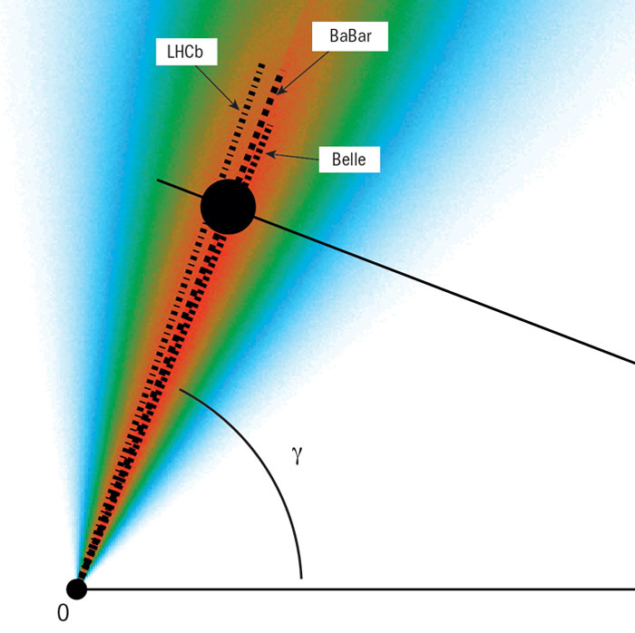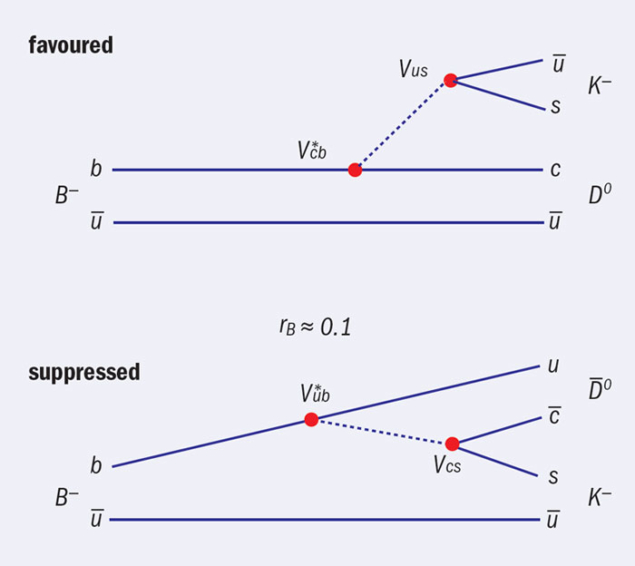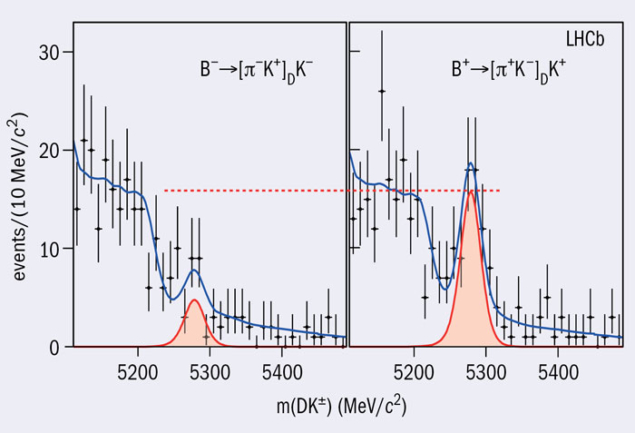# One CP-violating phase, three beautiful results

27 November 2012

Three experiments converge in their quest to measure γ.Fig. 1. An illustration of the three independent measurements of γ, as presented at CKM2012. The colouring gives an impression of the total uncertainty based on a combination of the three results. The large black dot indicates the expectation based on measurements of other Standard Model parameters.

The last day of September saw an exciting coincidence of three competing experiments simultaneously releasing three new and directly similar results. The occasion was the CKM2012 workshop in Cincinnati and the subject of interest: excellent new measurements of the CKM phase, γ.

Two of the contenders were well known to each other, having battled for supremacy in B physics for more than a decade. The “B factory” experiments, Belle and BaBar, were designed on the same principle: e+e collisions at the Υ(4S) resonance produce large numbers of BB pairs, which can be cleanly reconstructed in isolation. Except for a few selective technology choices, their most obvious dissimilarity is their location: Belle is at KEK in Japan while BaBar resides at SLAC in the US.

The meeting in Cincinnati saw these old foes joined by a new competitor, LHCb, which unlike the B factories collects its huge samples of bottom hadrons from high-energy proton–proton collisions at the LHC. Although there is little doubt that the CERN-based experiment will ultimately triumph with precision measurements of γ, on the morning of 30 September no one yet knew if that time had come.

Among the fundamental forces of nature, the weak force is special. Not only does it have a unique structure that gives rise to fascinating and often counter-intuitive physical effects, it is also highly predictive, making it excellent territory for searches for new physics. Perhaps the most celebrated phenomenon is CP violation – a common short-hand for saying that weak interactions of matter differ subtly from those of antimatter. Discovered in 1964 as a small effect (10–3) in KL0 decays, CP violation has more recently been observed as a large effect (10–2–10–1) in several B-meson decay modes.

### The CKM matrix

The size and variety of CP violation in b-quark transitions is widely acknowledged as a triumphant validation of the Cabibbo-Kobayashi-Maskawa (CKM) description of quarks coupling to W± bosons. This mechanism explains three-generation quark-mixing – up-type quarks (u, c, t) transmuting to and from down-type quarks (d, s, b) via the charged weak current – in terms of a 3 × 3 matrix rotation of the quarks’ mass eigenstates into their weak-interaction eigenstates. CP violation arises naturally through the mathematically mandatory presence of one complex phase in this generically complex matrix. Furthermore, if nature indeed has only three quark generations and probability is conserved, then the CKM transformation must be unitary.

Unitary matrices have a property that the scalar product of any two rows or columns must equate to zero. In the case of the 3 × 3 CKM matrix, six equations can be written down that must hold true if there are three – and only three – generations of quarks. Of these six relations, which are all triangles on the Argand plane, the most celebrated is

V*ubVud +V*cbVcd +V*tbVtd = 0

where each VXY is one of nine CKM matrix elements that encode the strength with which quark X couples to quark Y. This triangle, whose internal angles are usually labelled α, β and γ, is widely publicized because it summarizes concisely the largest CP-violating processes in B mesons. Studying the geometry of this unitarity triangle (UT) tests the internal consistency of the three-generation CKM picture of quark mixing. The lengths of the sides of the UT are measured in CP-conserving processes, whereas the size of the angles (or phases) can be measured only via CP-violating decays.

In Cincinnati, the BaBar collaboration announced that it had achieved a measurement of γ = 69+17–16° from a combination of many analyses of B± → D(*)K± decays. The precision of around 25% can be compared with the precision with which the other two UT angles are known. The smallest of the three angles, β, is known to less than 4%, β = 21.4 ± 0.8°, principally from measuring the time-dependent CP asymmetry in the mixing and decay of B→ J/ψK0 decays. The angle subtended by the apex of the triangle, α, is known to around 5%, α = 88.7+4.6–4.2°, from similar, time-dependent analyses of B0 → ππ and B0 → ρρ decays. Remembering that the three angles of a triangle always add up to 180°, it is clear that BaBar’s central value is remarkably close to the CKM expectation.

The Belle collaboration’s presentation quickly followed and explained a similar measurement of γ = 68+15–14°, the modest improvement perhaps being a result of the almost twice-as-large data set. As with BaBar, this number results from the careful combination of various measurements of CP-violating properties of B± → DK± and B± → D*K± decays.

### Interfering amplitudes

The B factories’ common choice of B± → DK± decays is not a coincidence. Among the current UT angle analyses, only γ measurements use direct CP violation in charged B decays. This promises a simple asymmetry of matter versus antimatter but requires two interfering amplitudes resulting in the same, indistinguishable final state. They must have different CP-conserving phases (generally true for any two quantum processes) and be of similar magnitude, or the influence of the less-likely process is too hard to detect.± → DK± decays” width=”700″ height=”624″> Fig. 2. The basic principle of accessing γ in B± → DK± decays. Two processes of similar magnitude are needed, one based on a b → c transition and the other b → u. These two paths must arrive at an indistinguishable final state such that phase information may be accessed via quantum interference.

In the UT definition, γ is identified as the weak phase difference between b → c and b → u quark transitions. Figure 2 shows Feynman diagrams for two paths of B± → DK±. The one involving a b → c quark transition is labelled “favoured” because a b quark is most likely to decay to a c quark. The second diagram involves a b → u quark transition and is labelled “suppressed” because the chance of its occurrence is around 1% of that of the favoured process (i.e. the ratio of amplitudes, rB is around 0.1).

This all looks good except for the detail in figure 2 that the favoured diagram results in a D0 while the suppressed diagram yields a D0. For the two B decays to interfere, the two neutral particles must be reconstructed in a final state that is common to both, i.e. the D0 and D0 should be indistinguishable. This might occur in the following ways, all of which are studied by Belle, BaBar and to some extent, LHCb.

• CP-eigenstate decays of neutral D mesons are by definition equally accessible to D0 and D0. In this case, the interference – and hence the size of the direct CP violation – is around 10% (from rB in figure 2). Examples of this type are B± → [K+K]DK± and B± → [KS0π0]DK± decays, where the D indicates that the particles in parentheses originated from a D meson.

• The unequal rate of the favoured and suppressed B decays can be redressed by selecting D final states that have an opposite suppression. Such combinations are referred to as ADS decays, after their original proponents. The most obvious example is B± → [π±K+–]DK± decays where, importantly, the kaon from the D decay is of an opposite charge to that emanating from the B decay. In this particular case, the favoured B decay from figure 2 is followed by the doubly Cabibbo-suppressed D0 → πK+ decay, whereas the suppressed B decay precedes a favoured D0 → K+π decay. With this opposite suppression, the total ratio of amplitudes (rB/rD) is much closer to unity than the first case, so larger CP violation, and hence greater sensitivity to γ, is achieved.

• A third possibility considers multi-body D decays such as B± → [KS0π+π]DK±. In this case, the kinematics of the three-body D decay is studied across a 2D histogram, the Dalitz plot. When the D → KS0π+π Dalitz plot for B → DK decays is compared with that of B+ → DK+ decays, they look identical except for a few places where γ has induced CP violation. Some places on the Dalitz plot have large sensitivity to γ, others less, but a big advantage comes from understanding the CP-conserving phases that vary smoothly across the Dalitz plot. Such an analysis is complicated, but worth it as the patterns of CP asymmetry across the Dalitz plane can be solved by only one value of γ (modulo 180°). This compares well to the first two cases whose interpretations suffer from trigonometric ambiguities because of their non-trivial sinusoidal dependence on γ.

Both the Belle and BaBar results combine all of these methods using B± → DK± and B± → D*K± decays. This diversity is vital since the branching fraction of γ-sensitive decays is so small (proportional to |Vub|2) and only a few hundred events have been collected in these experiments, even after a decade of operation.Fig. 3. Invariant mass distributions of ADS candidates from LHCb. The left plots are B– events, B+ events are on the right. The data, which were collected during 2011, are shown as black markers. The total fitted function is shown in blue and the signal, which exhibits a large asymmetry, is highlighted in red.

LHCb has different advantages and challenges. On one hand the huge cross-section for B production at the LHC means that LHCb has a considerable advantage in the number of charged-track-only decays that it can gather. On the other hand, because of the hadronic environment LHCb fairs less well with modes containing neutral particles. The D → KS0π+π mode is still useful, but cannot be relied on as heavily as at the B factories. Modes with a π0 or a photon, notably the otherwise important B± → D*K±, D* → D0π0/D0γ suite of modes, have not yet been attempted at LHCb.

Nevertheless for the charged-track final states, such as the easiest ADS modes, LHCb has triumphed with first observations of the B± → [π±K+–]DK± mode (see figure 3), as well as the similarly interesting B± → [π±K+–ππ+]DK± mode. By measuring the large CP asymmetries in these modes, and with the help of an ambiguity-busting B± → [KS0π+π]DK± analysis, the LHCb collaboration concluded the CKM2012 session by announcing a measurement of γ = (71.1+16.6–15.7)° from B± → DK± decays.

Such exotic processes are the reason for well established phenomena such as B-mixing and flavour-changing neutral-current decays

The simple combination of these three independent results (neglecting their common systematics) leads to the conclusion that γ is known to better than 14% accuracy: γ = 69.3+9.4–8.8°. This is illustrated in figure 1, which also shows the remarkable similarity of the three measurements and their mutual agreement with the expectation based on the world-average values of β and α.

The concluding theme in Cincinnati was that despite LHCb’s coming of age since CKM2010, the CKM description of the quarks’ weak interactions continues to prove impressively complete. It was noted however, that many flagship B-physics measurements, including the UT angles α and β, involve processes that contain quantum loops and/or boxes. Such exotic processes are the reason for well established phenomena such as B-mixing and flavour-changing neutral-current decays. Standard Model loop-processes contain the virtual existence of high-mass particles such as W±, top quarks and by extension, possibly non-Standard Model particles too. If they exist, and if they couple to quarks, such new-physics particles could be altering the physical behaviour of B mesons from the CKM-based expectation.

Detection of non-CKM effects is possible only if loop-sensitive observations can be compared with a gold-standard CKM process. B± → DK± decays provide exactly this. They are “tree-level” measurements (meaning, no loops) that are almost unique in heavy-flavour physics for their theoretical cleanliness. The measurement of γ in these modes is a measurement of γCKM, something the other two angles of the UT cannot boast with such certainty.

Though γ is currently the least well known UT property, by the end of this decade LHCb will have reduced its uncertainty to less than 5° (less than about 8%). By the end of the epoch of the Belle and LHCb upgrades, sub-degree precision looks likely. Such stunning precision will mean that this phase will become the CKM standard candle against which loop processes will be compared increasingly carefully.